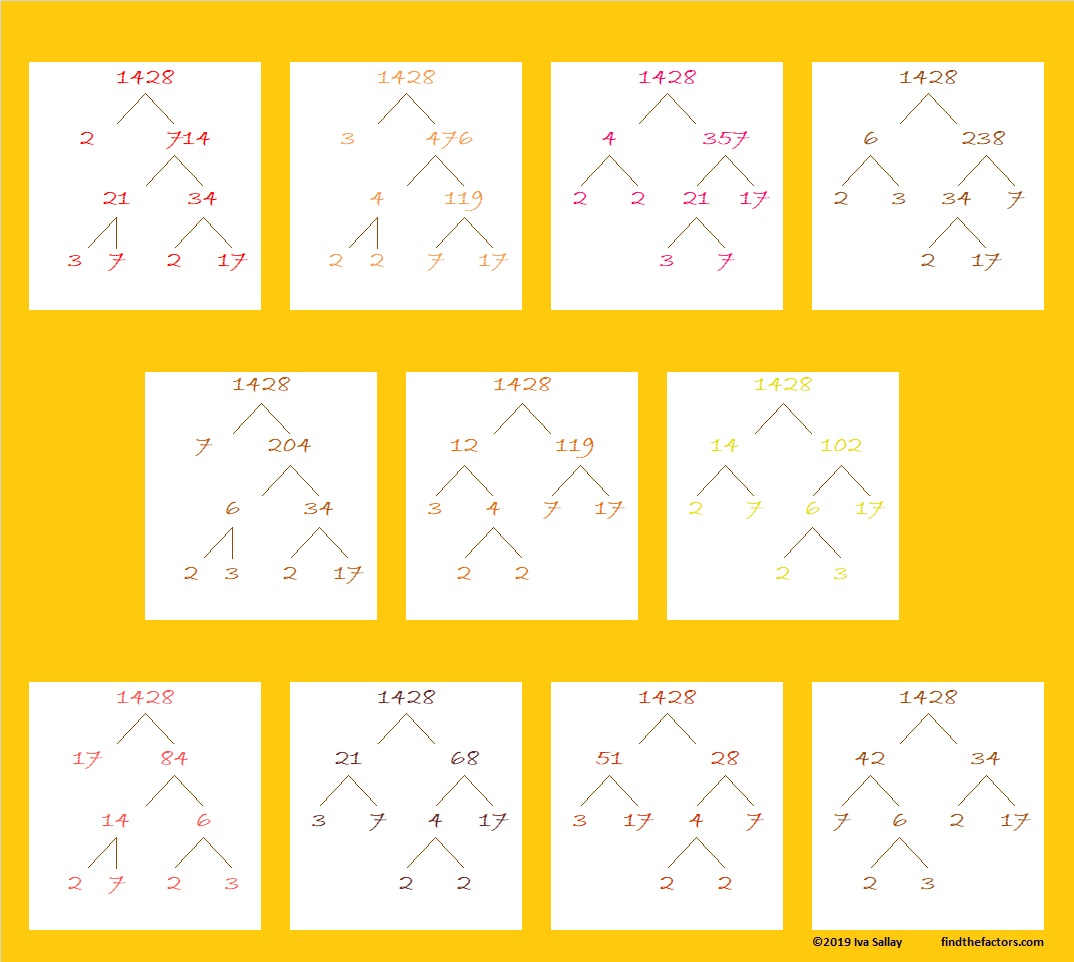# 1428 Factor Trees in Autumn

I recently decided that I wanted to make some factor trees in various fall colors. 1428 has plenty of factors so it has MANY different factor trees. Here are just eleven of them, each initially factored by a different factor pair.Here are some more facts about the number 1428:

• 1428 is a composite number.
• Prime factorization: 1428 = 2 × 2 × 3 × 7 × 17, which can be written 1428 = 2² × 3 × 7 × 17
• 1428 has at least one exponent greater than 1 in its prime factorization so √1428 can be simplified. Taking the factor pair from the factor pair table below with the largest square number factor, we get √1428 = (√4)(√357) = 2√357
• The exponents in the prime factorization are 2, 1, 1, and 1. Adding one to each exponent and multiplying we get (2 + 1)(1 + 1)(1 + 1)(1 + 1) = 3 × 2 × 2 × 2 = 24. Therefore 1428 has exactly 24 factors.
• The factors of 1428 are outlined with their factor pair partners in the graphic below.1428 is the hypotenuse of a Pythagorean triple:
672-1260-1428 which is (8-15-17) times 84.

This site uses Akismet to reduce spam. Learn how your comment data is processed.